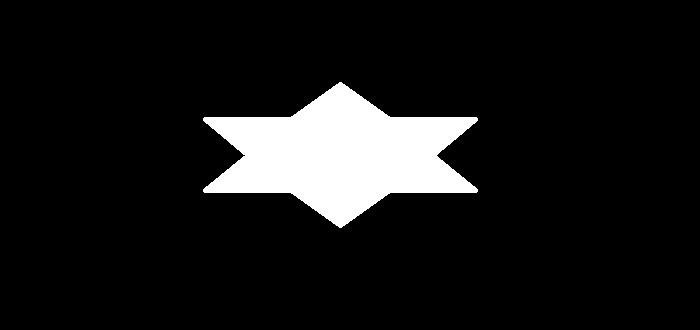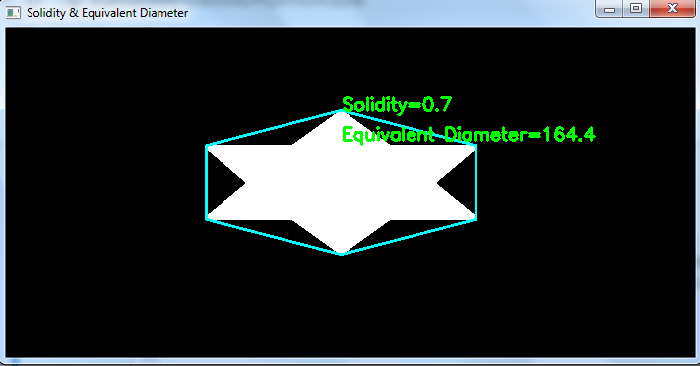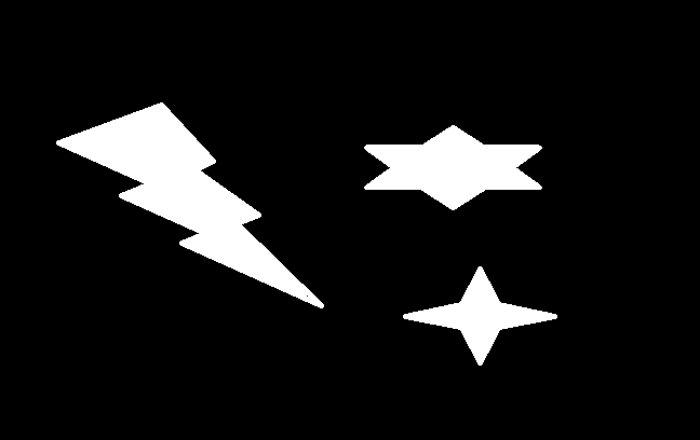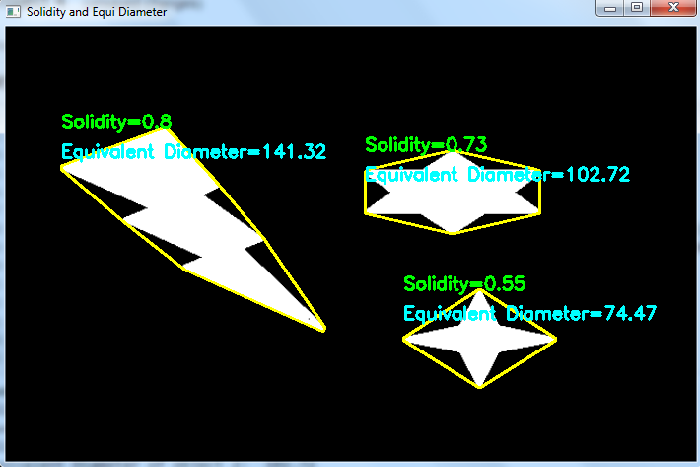# How to find the solidity and equivalent diameter of an object in an image using OpenCV Python?

The solidity of an object is computed as the ratio of contour area to its convex hull area. So to compute the solidity, we first have to find the contour area and convex hull area. The contour area of an object can be found using cv2.contourArea() function.

Equivalent Diameter is the diameter of the circle whose area is the same as the contour area. The solidity and equivalent diameter can be computed as below −

## Syntax

area = cv2.contourArea(cnt)
hull = cv2.convexHull(cnt)
hull_area = cv2.contourArea(hull)
solidity = float(area)/hull_area
equi_diameter = np.sqrt(4*area/np.pi)


Where, cnt is a numpy array of the contour points of an object in the image.

## Steps

You can use the following steps to compute solidity and equivalent diameter of an object in an image −

Import the required library. In all the following Python examples, the required Python library is OpenCV. Make sure you have already installed it.

import cv2


img = cv2.imread('star.png.png')
gray = cv2.cvtColor(img, cv2.COLOR_BGR2GRAY)


Apply thresholding on the grayscale image to create a binary image. Adjust the second argument for better detection of contour.

ret,thresh = cv2.threshold(gray,40,255,0)


Find the contours in the image using cv2.findContours() function.

contours, _ = cv2.findContours(thresh, cv2.RETR_TREE, cv2.CHAIN_APPROX_SIMPLE)


Select a contour "cnt" or loop over all contours. Compute the solidity and equivalent diameter of the object. We first compute the contour area and convex hull area.

area = cv2.contourArea(cnt)
hull = cv2.convexHull(cnt)
hull_area = cv2.contourArea(hull)
solidity = float(area)/hull_area
equi_diameter = np.sqrt(4*area/np.pi)


Optionally you can draw the Convex Hull on the input image. And put the solidity and equivalent diameter as text on the image

cv2.drawContours(img, [hull], -1, (0,255,255), 3)


Print the solidity and equivalent diameter and display the image with the drawn convex hull and written text.

print("Solidity: ", solid)
print("Equivalent Diameter: ", dia)
cv2.imshow("Solidity & Equivalent Diameter", img)
cv2.waitKey(0)
cv2.destroyAllWindows()


Let's have a look at some examples for more clear understanding.

## Example 1

In this Python program, we compute the solidity and equivalent diameter of an object in the image. We draw the convex hull for the object in the image. We also put on the image the solidity and equivalent diameter value as text for the object.

# import required libraries
import cv2

# convert the image to grayscale
gray = cv2.cvtColor(img, cv2.COLOR_BGR2GRAY)

# apply thresholding to convert grayscale to binary image
ret,thresh = cv2.threshold(gray,40,255,0)

# find the contours
contours,hierarchy = cv2.findContours(thresh, cv2.RETR_TREE,cv2.CHAIN_APPROX_SIMPLE)
print("Number of objects detected:", len(contours))

# define function to compute the solidity
def solidity(cnt):
area = cv2.contourArea(cnt)
hull = cv2.convexHull(cnt)
hull_area = cv2.contourArea(hull)
solidity = float(area)/hull_area
return solidity

# define function to compute the equivalent diameter
def eq_dia(cnt):
area = cv2.contourArea(cnt)
equi_diameter = np.sqrt(4*area/np.pi)
return equi_diameter

# select first contour
cnt = contours

# find the solidity for this contour
solid = solidity(cnt)
solid= round(solid, 2)

# find the equivalent diameter for this contour
dia = eq_dia(cnt)
dia =round(dia, 2)

# draw convex hull on the image
hull = cv2.convexHull(cnt)
x1, y1 = hull
img = cv2.drawContours(img,[hull],0,(255,255,0),2)

cv2.putText(img, f'Solidity={solid}', (x1, y1), cv2.FONT_HERSHEY_SIMPLEX, 0.6, (0, 255, 0), 2)
cv2.putText(img, f'Equivalent Diameter={dia}', (x1, y1+30), cv2.FONT_HERSHEY_SIMPLEX, 0.6, (0, 255, 0), 2)

print("Solidity: ", solid)
print("Equivalent Diameter: ", dia)

cv2.imshow("Solidity & Equivalent Diameter", img)
cv2.waitKey(0)
cv2.destroyAllWindows()


We will use this image as the Input File in this program −## Output

When we execute the above code, it will produce the following output −

Number of objects detected: 1
Solidity: 0.7
Equivalent Diameter: 164.4


And we get the following output window −The output shows the detected object and convex hull is drawn in cyan color. The solidity and equivalent diameter of the detected object is written in green color.

## Example 2

In this Python program, we compute the solidity and Equivalent diameter of multiple objects in the image. We draw the convex hull for each objects on the image. We also put the solidity and equivalent diameter as text for each object.

import cv2
import numpy as np

gray = cv2.cvtColor(img, cv2.COLOR_BGR2GRAY)

ret,thresh = cv2.threshold(gray,100,255,0)
contours,hierarchy = cv2.findContours(thresh, 1, 2)
print("Number of objects detected:", len(contours))

def solidity(cnt):
area = cv2.contourArea(cnt)
hull = cv2.convexHull(cnt)
hull_area = cv2.contourArea(hull)
solidity = float(area)/hull_area
return solidity

def eq_dia(cnt):
area = cv2.contourArea(cnt)
equi_diameter = np.sqrt(4*area/np.pi)
return equi_diameter

for i, cnt in enumerate(contours):
solid = solidity(cnt)
solid= round(solid, 2)
dia = eq_dia(cnt)
dia =round(dia, 2)
x,y,w,h = cv2.boundingRect(cnt)
hull = cv2.convexHull(cnt)
img = cv2.drawContours(img,[hull],0,(0,255,255),2)
cv2.putText(img, f'Solidity={solid}', (x, y), cv2.FONT_HERSHEY_SIMPLEX, 0.6, (0, 255, 0), 2)
cv2.putText(img, f'Equivalent Diameter={dia}', (x, y+30), cv2.FONT_HERSHEY_SIMPLEX, 0.6, (255, 255, 0), 2)
print(f"Solidity of object {i+1}: ", solid)
print(f"Equivalent Diameter of object {i+1}: ", dia)

cv2.imshow("Solidity and Equi Diameter", img)
cv2.waitKey(0)
cv2.destroyAllWindows()


We will use this image as the Input File in this program −## Output

When we execute the above code, it will produce the following output

Number of objects detected: 3
Solidity of object 1: 0.55
Equivalent Diameter of object 1: 74.47
Solidity of object 2: 0.73
Equivalent Diameter of object 2: 102.72
Solidity of object 3: 0.8
Equivalent Diameter of object 3: 141.32


And we get the following output window −The convex hull is drawn in yellow color. It also shows the solidity and equivalent diameters for each object.

Updated on: 28-Sep-2022

806 Views